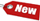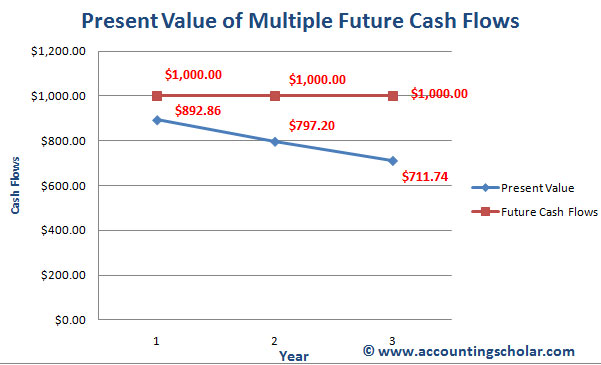Browse Accounting Lessons HereAccounting Terms & DefinitionsAccounting for Merchandising ActivitiesDebits and Credits (Double Entry Accounting)Business Valuation FormulasTime Value of Money & Present/Future ValuesComplex Debt & Equity InstrumentsCommon Stock & Shareholder's EquityAccounting & Finance RatiosValuing Common StockCorporate Income TaxesLower of Cost or Market (LCM) & Inventory ValuationChart of Accounts & BookkeepingBonds Payable & Long Term LiabilitiesCapital AssetsGAAP, Accrual & Cash Accounting, Information Commodity, Internal Controls & MaterialityWhat category of browser are you on this website? Accounting student (homework help) Finance professor (university research) Accounting manager (at work) Other Explore Careers in Accounting and FinanceVisit our section on Careers in Accounting & Finance to explore vast opportunities in this industry.

Chapter 4.15® - Valuing Annuity Level Cash Flows – Present & Future Value of Annuities, Find Present Value of \$400 at Year 2, 9% rate of return

So far we have looked at present & future value of multiple cash flows in the future that could be of different sizes, payment frequencies and with different interest rates. In the world of annuities however, this changes slightly as an annuity is income from a capital investment that is paid in a series of regular payments e.g. quarterly, semi-annual or annual payments. An example of annuity is a loan repayment plan that calls for the borrower to repay the loan by making a series of equal payments over some length of time. Examples of such payments include car payments, student loan payments or mortgage payments. A series of constant or equal cash flows that occur at the end of each period for some fixed number of periods is called an ordinary annuity.

Present Value for Annuity Cash Flows

Say we have an investment that promised to pay us \$1000 at the end of each of the next three years. The cash flows from this investment are in the form of a three year, \$1000 ordinary annuity. If we would like to earn a 12% return on our money, what is the most we should pay for this investment? In other words, what is the present value of this investment as of now?

We know we can calculate this using the present value discount factor for cash flows.

 Present Value = \$1 x [1/ (1 + r)t] = \$1/ (1 + r)t Present Value = (\$1000 / 1.121) + (\$1000 / 1.122) + (\$1000 / 1.123) Present Value = (\$1000 / 1.12) + (\$1000 / 1.2544) + (\$1000 / 1.405) Present Value = \$892.86 + \$797.20 + \$711.744 Present Value = \$2,401.804

 Years Present Value Future Cash Flows 1 \$892.86 \$1,000.00 2 \$797.20 \$1,000.00 3 \$711.74 \$1,000.00 Total: \$2,401.80 \$3,000.00This graph above shows the present value of each of the \$1,000 annuity payments with the PV being \$892.86 in year 1, \$797.20 in year 2 and \$711.74 in year 3; notice as the years go by, the present value drops.

This approach works fine when the number of periods is as little as 3 – 5 periods. However, most financial analysts encounter complicated problems in which the number of cash flows & periods is quite large; an example includes a typical home mortgage that has monthly payments over 25 years, or a total of 300 payments. If we tried to calculate the present value of a mortgage using our equation method, it would be super time consuming and not efficient. However, we do have a shortcut formula which will help in this scenario.

Because the cash flows of an annuity are the same throughout its life, we can derive a very useful variation of the basic present value factor equation. We derive that the present value of an annuity of C dollars per period for t periods when the rate of return or interest rate is r is given by:

 Annuity Present Value = C x [(1 – Present Value Factor) / r] Annuity Present Value = C x [(1 – 1/(1+r)t) / r]

The term in parentheses is known as the present value discount factor and is derived from:

 Present Value = \$1 x [1/ (1 + r)t] = \$1/ (1 + r)t

So let’s revisit the problem we addressed above using this annuity present value formula. Say we have an investment that promised to pay us \$1000 at the end of each of the next three years. The cash flows from this investment are in the form of a three year, \$1000 ordinary annuity. If we would like to earn a 12% return on our money, what is the most we should pay for this investment? In other words, what is the present value of this investment as of now?

 Present value factor = \$1/ (1 + r)t Present value factor = 1 / (1 + 0.12)3 Present value factor = 1 / (1.12)3 Present value factor = 1 / 1.405 Present value factor = 0.712

To calculate the annuity present value factor, we must plug in the numbers:

 Annuity present value factor = (1 – present value factor) / r Annuity present value factor = (1 – 0.712) / 0.12 Annuity present value factor = 0.288 / 0.12 Annuity present value factor = 2.4 Present value of Annuity = 2.4 times x \$1,000 = \$2,400

Notice this \$2,400 is very close to the manual answer we calculate above of \$2401.804, the difference is due to rounding issues.

Can we do these calculations using our BAII plus financial calculator? We sure can! To find annuity present values with a financial calculator, we need to use the PMT key in the calculator.
Note: We do not enter anything for the FV field, and instead enter the PMT function.

i) Find Present Value of \$400 at Year 2, 9% rate of return.

 N = 3 I/Y = 12% PV = ? PMT = \$1,000 FV = \$0 2ND I/Y P/Y = 1 C/Y = 1 CPT & PV = \$2,401.83